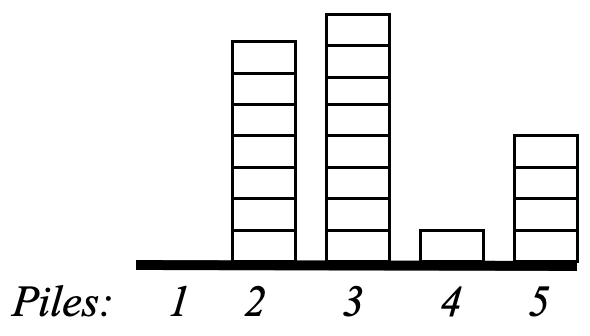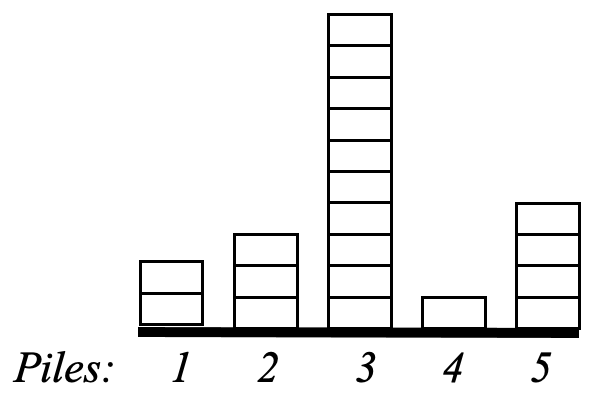시간 제한메모리 제한제출정답맞힌 사람정답 비율
1 초 128 MB1000.000%

## 문제

A solitaire game is played given a row of N piles where each pile contains zero or more chips. See Figure 1. Piles are identified by integers 1 through N. In a move of this game you point to a pile, say p, and specify a number, say m. Then m chips are transferred from the pile p to each of its neighboring piles. See Figure 2 for an example. Pile p has two neighbors, p-1 and p+1 if 1<p<N, and the neighbor 2 if p=1, and the neighbor N-1 if p=N. Note that to be able to make such a move the pile p must have at least 2m chips if it has two neighbors, and it must have at least m chips if it has only one neighbor.

The object of the game is to "flatten" all piles, that is make them have the same number of chips, by making as small as possible number of moves. In case there is more than one solution you are required to report only one of them.Figure 1. Five piles with 0, 7, 8, 1 and 4 chips. Figure 2. Same piles after a move: p=2, m=2

## 입력

• The first line: N
• The second line: there will be N integers the ith of which is the value of Ci.

## 출력

• The first line: number of moves. (Call this value M.)
• Each of the following M lines contains two integers representing a move: p, m.

The order of moves on the output must be the same order the moves are performed. So, your first move shall be written to the second line of the output file.

## 제한

• It is guaranteed that it is possible to flatten the given piles in at most 10,000 moves.
• 2 ≤ N ≤ 200
• 0 ≤ Ci ≤ 2000 where Ci is the number of chips in the pile i when the game starts (1 ≤ iN).

## 예제 입력 1

5
0 7 8 1 4


## 예제 출력 1

5
5 2
3 4
2 4
3 1
4 2